Solving by Combining Like Terms
Solving by Using the Distributive Property
Expressions
Equation Grab Bag
Word Problems
100
5h + 2 + 2h = 23
h = 3
100
2(x + 5) = 14
x = 2
100Somewhere over the Rainbow

100Wave goodbye

100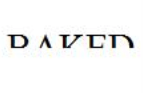Half Baked

200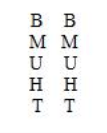Thumbs Up

200

Troy has more than two dogs at home. All of them are corgis, except for two. All of them are pugs, except for two. All of them are labs, except for two. What kinds of dogs and how many of each kind does Troy have?

3 Dogs: one corgi, one pug, one lab

200
Simplify. 19x - 24x
-5x
200
x + 3x = -8
x = -2
200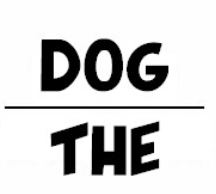The underdog

300
3a + 12 - 6a = -9
a = 7
300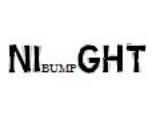Bump in the Night

300
Simplify. 3 + 5(a + 4)
5a + 23
300

What do an island and the letter “t” have in common?

They are both in the middle of water.

300
Tyler has 4 boxes of apples. Each box has the same number of apples. After Tyler eats 3 apples, there are 109 apples left in the boxes. How many apples were in each box?
a - # of apples in each box 4a - 3 = 109 a = 28 There were 28 apples in each box.
400

A father and son are in a horrible car crash that kills the dad. The son is rushed to the hospital; just as he’s about to go under the knife, the surgeon says, “I can’t operate—that boy is my son!”

Who is the surgeon?

The boy's mother
400
-2(x - 9) = -24
x = 21
400
Simplify. - (2b - 4)
-2b + 4
400
-6(m + 1) + 18 = 24
m = -2
400
Mr. Bucci goes to the grocery store and purchases 17 bags of raisins and a \$2.97 jar of peanut butter. His total cost was \$34.76. What was the cost for a bag of raisins?
r - cost of a bag of raisins 17r + 2.97 = 34.76 r = 1.87 Each bag of raisins cost \$1.87
500
78 = 3c + 12 - c + 4
c = 31
500
-13(4 + 2a) = 208
a = -10
500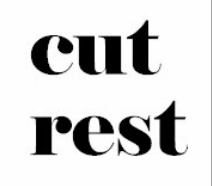Cut above the rest

500
7k - 8 + 2(k + 12) = 52
k = 4
500

What runs but never walks,

has a mouth but never talks,

has a bed but never sleeps,

has a head but never weeps?

A river

Click to zoom